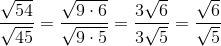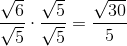# PSAT Math : How to divide square roots

## Example Questions

### Example Question #1 : How To Divide Square Roots

Divide and simplify. Assume all integers are positive real numbers.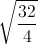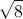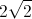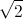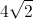Explanation:There are two ways to solve this problem. First you can divide the numbers under the radical. Then simplify.

Example 1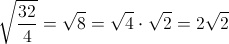Example 2

Find the square root of both numerator and denominator, simplifying as much as possible then dividing out like terms.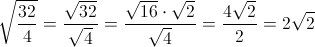Both methods will give you the correct answer of.

### Example Question #1 : How To Divide Square Roots

(√27 + √12) / √3 is equal to

5/√3

5

(6√3)/√3

18

√3

5

Explanation:

√27 is the same as 3√3, while √12 is the same as 2√3.

3√3 + 2√3 = 5√3

(5√3)/(√3) = 5

### Example Question #2 : How To Divide Square Roots

Simplify: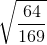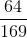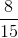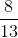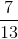Explanation:

To simplfy, we must first distribute the square root.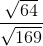Next, we can simplify each of the square roots.### Example Question #3 : How To Divide Square Roots

Find the quotient: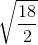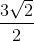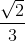Explanation:

Find the quotient: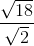There are two ways to approach this problem.

Option 1: Combine the radicals first, the reduce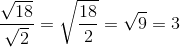Option 2: Simplify the radicals first, then reduce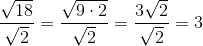### Example Question #4 : How To Divide Square Roots

Find the quotient: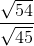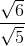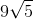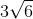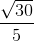Explanation: